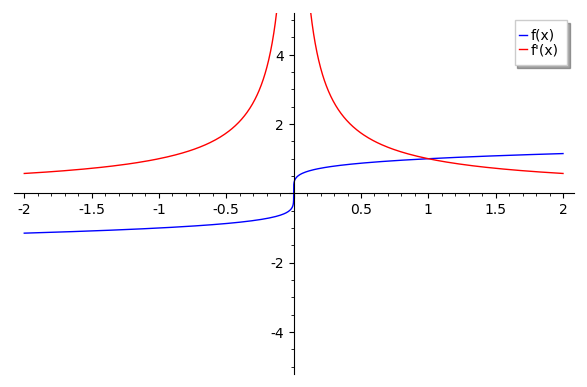# Problem 1¶

Let $h(x) = (x^4 - 2) f(x)$, $f(2) = 2$, and $f'(2) = 4$. Then what is $h'(2)$?

We can write $h(x) = f(x) g(x)$, where $g(x) = x^4 - 2$. By the product rule, we have that

$h'(x) = f(x)g'(x) + g(x)f'(x)$

and we compute $g'(x) = 4x^3$

Therefore, $h'(x) = 4x^3f(x) + (x^4-2)f'(x)$

And $h'(2) = 4*2^3*f(2) + (2^4-2)*f'(2) = 32*2 + 14*4 = 120$

# Problem 2¶

Let $f(x) = x^{1/5}$. What is $f'(x)$? Where is $f'(x)$ discontinous? What is the range of $f'(x)$?

By the power rule, $\Large f'(x) = \frac{1}{5}x^{-4/5} = \frac{1}{\sqrt{x^4}}$

From this, we see that we have x raised to an even power in the denominator. This means that $f'(x)$ is always positive. Furthermore, $\lim_{x \to +/- \infty} f'(x) = 0$, but it never quite reaches $0$. So the range is $(0, \infty)$.

When $x = 0$, we end up with a continuity problem, because we have $1/0$. The function is plotted below with its derivative. Notice how the derivative is always positive, but it blows up at zero, and its limit is zero in either direction

In :
# This is just a technicality with code, but we have to do something annoying
# to get around the fact that Sage returns complex numbers for negative inputs
# rasied to fractions
f = sign(x)*abs(x)^(1/5)
fprime = 1/((x^4)**0.2)

In :
p1 = plot(f, (x, -2, 2), ymin=-5, ymax=5, legend_label="f(x)")
p2 = plot(fprime, (x, -2, 2), ymin=-5, ymax=5, color='red', legend_label="f'(x)")
show(p1+p2)# Problem 3¶

Let $f(x) = 2x^6 - 4x^2 - \sqrt{x}$. Then what is f'(x)?

We apply the power rule, constant multiple rule, and sum rules all at once to obtain

$f'(x) = 12x^5 - 8x - \frac{1}{2 \sqrt{x}}$

# Problem 4¶

Evaluate the following limit and show your work

$\Large \lim_{h \to 0} \frac{(5+h)^2 - 25}{h}$

Find an $f(x)$ and an $a$ so that the limit represents $f'(a)$

We can expand the numerator to obtain

$\Large \lim_{h \to 0} \frac{25 + 10h + h^2 - 25}{h}$

then we combine like terms to get

$\Large \lim_{h \to 0} \frac{10h + h^2}{h} = \lim_{h \to 0} 10 + h = 10$

We notice that by the definition of the derivative, this is actually the derivative of $f(x) = x^2$ at $a = 5$

In [ ]: